# Similarity Py

## Installation

Install the package

`    \$ pip install similarityPy`

## Dependencies

``````enum
``````

### Distance Algorithms

#### Numerical Data

##### Norm

Data: [{x, y, z}]
Formula: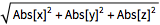##### Manhattan Distance

Data: [{a, b, c}, {x, y, z}]
Formula: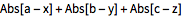##### Euclidean Distance

Data: [{a, b, c}, {x, y, z}]
Formula: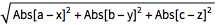##### Squared Euclidean Distance

Data: [{a, b, c}, {x, y, z}]
Formula: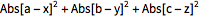##### Normalized Squared Euclidean Distance

Data: [{a, b}, {x, y}]
Formula: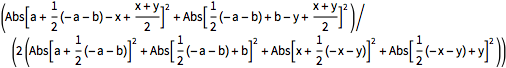##### Chessboard Distance

Data: [{a, b, c}, {x, y, z}]
Formula: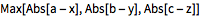##### Bray Curtis Distance

Data: [{a, b, c}, {x, y, z}]
Formula: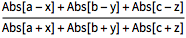##### Canberra Distance

Data: [{a, b, c}, {x, y, z}]
Formula: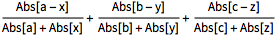##### Cosine Distance

Data: [{a, b, c}, {x, y, z}]
Formula: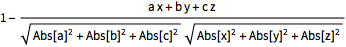##### Correlation Distance

Data: [{a, b, c}, {x, y, z}]
Formula: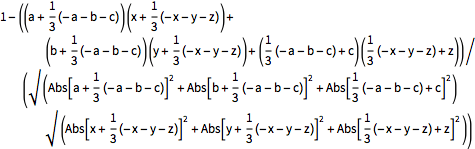#### Boolean Data

##### Jaccard Dissimilarity

Data: [{True,False,True}, {True,True,False}]
Explanation:[u,v] is equivalent to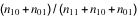, where nij is the number of corresponding pairs of elements in u and v respectively equal to i and j.

##### Matching Dissimilarity

Data: [{True,False,True}, {True,True,False}]
Explanation:[u,v] is equivalent to (n10+n01)/Length[u], where nij is the number of corresponding pairs of elements in u and v respectively equal to i and j.

##### Dice Dissimilarity

Data: [{True,False,True}, {True,True,False}]
Explanation:[u,v] is equivalent to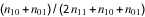, where nij is the number of corresponding pairs of elements in u and v respectively equal to i and j.

##### Rogers Tanimoto Dissimilarity

Data: [{True,False,True}, {True,True,False}]
Explanation:[u,v] is equivalent to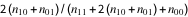, where nij is the number of corresponding pairs of elements in u and v respectively equal to i and j.

##### Russell Rao Dissimilarity

Data: [{True,False,True}, {True,True,False}]
Explanation:[u,v] is equivalent to (n10+n01+n00)/Length[u], where nij is the number of corresponding pairs of elements in u and v respectively equal to i and j.

##### Sokal Sneath Dissimilarity

Data: [{True,False,True}, {True,True,False}]
Explanation:[u,v] is equivalent to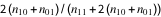, where nij is the number of corresponding pairs of elements in and respectively equal to i and j.

##### Yule Dissimilarity

Data: [{True,False,True}, {True,True,False}]
Explanation:[u,v] is equivalent to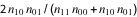, where nij is the number of corresponding pairs of elements in and respectively equal to i and j.

#### String Data

##### Hamming Distance

Data: [{a, b, c}, {x, y, z}]
Explanation:[u,v] gives the number of elements whose values disagree in u and v.

##### Edit Distance

Data: [{a, b, c}, {x, y, z}]
Explanation:[u,v] gives the number of one-element deletions, insertions, and substitutions required to transform u to v.

##### Damerau Levenshtein Distance

Data: [{a, b, c}, {x, y, z}]
Explanation:[u,v] gives the number of one-element deletions, insertions, substitutions, and transpositions required to transform u to v.

##### Needleman Wunsch Similarity (Not Implemented Yet)

Data: [{a, b, c}, {x, y, z}]
Explanation:[u,v] finds an optimal global alignment between the elements of u and v, and returns the number of one-element matches.

##### Smith Waterman Similarity (Not Implemented Yet)

Data: [{a, b, c}, {x, y, z}]
Explanation:[u,v] finds an optimal local alignment between the elements of u and v, and returns the number of one-element matches.

## Testing

Run all tests:

`    \$ python -m unittest discover -s tests -p '*_test.py'`

Start test with nose and code coverage:

`    \$ nosetests --with-cov  --cov-report html  --cov  similarityPy tests/`